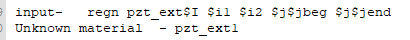# what is wrong with my material code? Answered

hey,

I want to define several pzt materials by matr command.My defined pzt materials have same property but different material name.I used ‘do loopI’ to define different material name ,like the following material code.But when I assigned the pzt materials with different material name to different area of my model，the debug window says unknown material. Is it wrong to name the material in this way？And how to define the material with same property but different name？

By the way ，I have defined  the variable ‘ narelem’.

Best regards1. the material code
`axisform vctrdefn posx car2 0. 0. 0. 1. 0. 0. 0. 1. 0.defn negx car2 0. 0. 0. -1. 0. 0. 0. 1. 0.defn posy car2 0. 0. 0. 0. 1. 0. 0. 0. 1.defn negy car2 0. 0. 0. 0. -1. 0. 0. 0. 1.defn posz car2 0. 0. 0. 0. 0. 1. 1. 0. 0.defn negz car2 0. 0. 0. 0. 0. -1. 1. 0. 0.endc -------------------------------------------------------------------------c Input material properties to programc -------------------------------------------------------------------------matr c -------------------------------------------------------------- c type : PIEZO : c name : pzt: c desc : pzt Generic : c --------------------------------------------------------------c define baseline dielectric coefficientssymb epxx = 916 /* dielectric constant (constant strain)symb epyy = 916 /* dielectric constant (constant strain)symb epzz = 830 /* dielectric constant (constant strain)symb rho = 7750 /* densitysymb qdmp = 75 /* Mechanical Q at 1e6symb qsdmp = 75 /* Mechanical Q at 1e6symb freqdamp = 1.e6 if noexistsymb freqloss = 1e+06 if noexist /* Frequency that loss was measured atc define baseline stiffness coefficientssymb c11 = 1.203e+11 /* stiffness constant (constant electric field)symb c12 = 7.52e+10 /* stiffness constantsymb c13 = 7.51e+10 /* stiffness constantsymb c14 = 0 /* stiffness constantsymb c15 = 0 /* stiffness constantsymb c16 = 0 /* stiffness constantsymb c22 = 1.203e+11 /* stiffness constant (constant electric field)symb c23 = 7.51e+10 /* stiffness constantsymb c24 = 0 /* stiffness constantsymb c25 = 0 /* stiffness constantsymb c26 = 0 /* stiffness constantsymb c33 = 1.109e+11 /* stiffness constantsymb c34 = 0 /* stiffness constantsymb c35 = 0 /* stiffness constantsymb c36 = 0 /* stiffness constantsymb c44 = 2.11e+10 /* stiffness constantsymb c45 = 0 /* stiffness constantsymb c46 = 0 /* stiffness constantsymb c55 = 2.11e+10 /* stiffness constantsymb c56 = 0 /* stiffness constantsymb c66 = 2.26e+10c define baseline piezoelectric coupling coefficientssymb ex1 = 0 /* coupling constantsymb ex2 = 0 /* coupling constantsymb ex3 = 0 /* coupling constantsymb ex4 = 0 /* coupling constantsymb ex5 = 12.3 /* coupling constantsymb ex6 = 0 /* coupling constantsymb ey1 = 0 /* coupling constantsymb ey2 = 0 /* coupling constantsymb ey3 = 0 /* coupling constantsymb ey4 = 12.3 /* coupling constantsymb ey5 = 0 /* coupling constantsymb ey6 = 0 /* coupling constantsymb ez1 = -5.4 /* coupling constantsymb ez2 = -5.4 /* coupling constantsymb ez3 = 15.8 /* coupling constantsymb ez4 = 0 /* coupling constantsymb ez5 = 0 /* coupling constantsymb ez6 = 0 /* coupling constantc scale material properties as specified abovesymb aepxx = \$epvacm * \$epxxsymb aepyy = \$epvacm * \$epyysymb aepzz = \$epvacm * \$epzzwvsp off type leando loopI I \$narelem 1prop pzt_ext\$I \$rho\$c11 \$c12 \$c13 \$c14 \$c15 \$c16 \$c22\$c23 \$c24 \$c25 \$c26 \$c33 \$c34 \$c35\$c36 \$c44 \$c45 \$c46 \$c55 \$c56 \$c66elec pzt_ext\$I \$aepxx \$aepyy \$aepzzpiez pzt_ext\$I 1 1 \$ex1 1 2 \$ex2 1 3 \$ex3 1 4 \$ex4 1 5 \$ex5 1 6 \$ex6 &2 1 \$ey1 2 2 \$ey2 2 3 \$ey3 2 4 \$ey4 2 5 \$ey5 2 6 \$ey6 &3 1 \$ez1 3 2 \$ez2 3 3 \$ez3 3 4 \$ez4 3 5 \$ez5 3 6 \$ez6rdmp pzt_ext\$I \$freqdamp q \$qdmp \$qsdmp \$freqloss 1.0axis pzt_ext\$I posx /* relate materials local system to global systemend\$ loopIend`

2. site material to the model

` do loopI I 1 \$narelem 1symb jend = 2 * \$I + 1symb jbeg = \$jend - 1regn pzt_ext\$I \$i1 \$i2 \$j\$jbeg \$j\$jendend\$ loopI`

`do loop I 1 \$narelem 1 copy pzt_ext pzt_ext\$Iend\$ loop`